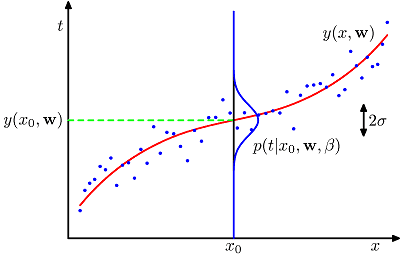# Basic notation (conditional probability delim in linear equation)

Hey all.

Looking at "Pattern Recognition and Machine Learning" (Bishop, 2006) p28-31, the author appears to be using what would ordinarily be a delimiter for a conditional probability inside a linear function. See the first variable in normpdf as below. This is in the context of defining a Bayesian prior distribution over polynomial coefficients in a curve fitting problem.

$$p(\textbf{w} | \alpha) = NormPDF(\textbf{w} | \textbf{0}, \alpha^{-1}\textbf{I}) = \left(\frac{\alpha}{2\pi}\right)^{(M+1)/2} exp \left(-\frac{\alpha}{2}\textbf{w}^T\textbf{w}\right)$$

Can anybody shine some light on this for me please?

Many thanks.

## Answers and Replies

CompuChip
Science Advisor
Homework Helper
I don't know if this is precisely the case here, but sometimes delimiters other than comma are used in functions. I have mostly seen semicolons (;) and vertical bars (|).
Often this is done to separate arguments by meaning. For example, an author may write
Consider a normal distribution with mean $\mu$ and standard deviation $\sigma$. We define the probability of finding a value between a and b as $$P(a, b \mid \mu, \sigma)$$ as ...
You can just as well write $$P(x, \mu, \sigma)$$. However, writing a separate delimiter hopefully makes it more clear to the reader that a and b are really the variables here and, though technically mu and sigma are variables as well, in this case they are more like parameters that have been previously fixed (some arbitrary values for some normal distribution we are interested in).

I don't know if this is precisely the case here, but sometimes delimiters other than comma are used in functions. I have mostly seen semicolons (;) and vertical bars (|).
Often this is done to separate arguments by meaning. For example, an author may write

You can just as well write $$P(x, \mu, \sigma)$$. However, writing a separate delimiter hopefully makes it more clear to the reader that a and b are really the variables here and, though technically mu and sigma are variables as well, in this case they are more like parameters that have been previously fixed (some arbitrary values for some normal distribution we are interested in).

Thanks for your reply.

Although I am quite sure that's not the case in this particular instance, in general, I know non-variable parameters may be written after a semicolon.

I believe the case to be that it reads as, "the value of $$t_n$$ evaluated for $$y(x_n,\textbf{w})$$" as described on http://en.wikipedia.org/wiki/Vertical_bar#Mathematics".

Elsewhere the likelihood of the parameters $$\{w,\beta\}$$ is written for two i.i.d. variables $$\{\textbf{x,t}\}$$ where the function y(x,w) computes the predicted value of t.

$$p(\textbf{t}|\textbf{x},w,\beta) = \prod_{n=1}^N NormPDF(t_n|y(x_n, \textbf{w}),\beta^{-1})$$So it seams a reasonable interpretation in this context.

Last edited by a moderator:
Hey all.

This is in the context of defining a Bayesian prior distribution over polynomial coefficients in a curve fitting problem.

$$p(\textbf{w} | \alpha) = NormPDF(\textbf{w} | \textbf{0}, \alpha^{-1}\textbf{I}) = \left(\frac{\alpha}{2\pi}\right)^{(M+1)/2} exp \left(-\frac{\alpha}{2}\textbf{w}^T\textbf{w}\right)$$

Can anybody shine some light on this for me please?

Many thanks.

I don't know what this is. The Bayesian expression for the conditional probability p(w|a) is:

p(w|a)=p(a|w)p(w)/p(a).

I don't know what this is. The Bayesian expression for the conditional probability p(w|a) is:

p(w|a)=p(a|w)p(w)/p(a).

Well there're a bit more to it. The formula you quoted is just for the prior.

The derivation is thus.

$$p(w|x,t,\alpha,\beta)$$ = (likelihood * prior) / marginal likelihood

$$p(w|x,t,\alpha,\beta) \propto p(t|x,w,\beta) * p(w|\alpha)$$

$$\{\alpha,\beta\}$$ are hyperparameters.

Well there're a bit more to it. The formula you quoted is just for the prior.

The derivation is thus.

$$p(w|x,t,\alpha,\beta)$$ = (likelihood * prior) / marginal likelihood

$$p(w|x,t,\alpha,\beta) \propto p(t|x,w,\beta) * p(w|\alpha)$$

$$\{\alpha,\beta\}$$ are hyperparameters.

OK. I was going by the original equation where the left side was simply $$p($$w$$|\alpha)=$$